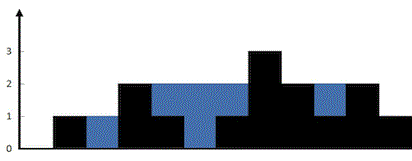bolt
Try our new interface for solving problems
Problems

# Trapping Rain Water

Time limit 1 second
Memory limit 128 MiB

Given n non-negative integers representing an elevation map where the width of each bar is 1.

Compute how much water it can trap after raining.## Input data

The first line contains the value of n~(n \le 10^5).

The second line contains n non-negative integers h_1, h_2, ..., h_n~(h_i \le 10^5).

## Output data

Print how much water can be trapped after raining.

## Examples

Input example #1
12
0 1 0 2 1 0 1 3 2 1 2 1

Output example #1
6

Input example #2
6
4 2 0 3 2 5

Output example #2
9